# 快速排序 Quicksort

Quicksort是一种分而治之的算法。它通过从数组中选择一个“pivot”元素并将其他元素划分为两个子数组（根据它们是否小于或大于基准数）来工作。然后将子数组递归排序。这可以就地完成，需要少量额外的内存来执行排序。

# 算法流程

Quicksort是一种分而治之的算法。

1. 从数组中选择一个称为基准数的元素。

2. 分区：对数组重新排序，以使所有值小于基准数的元素都位于基准数之前，而所有值大于基准数的元素都位于基准数之后（相等的值可以任意选择）。分割之后，基准数处于其最终位置。这称为分区操作。

3. 将上述步骤递归地应用于值较小的元素子数组，并分别应用于值较大的元素的子数组。

# 例子

## 分区

### 第一次交换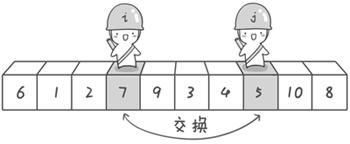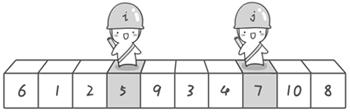### 第二次交换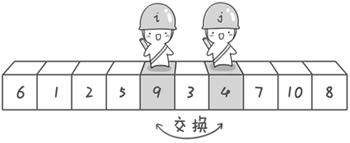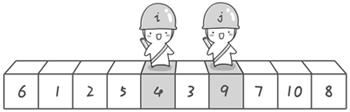### 第三次交换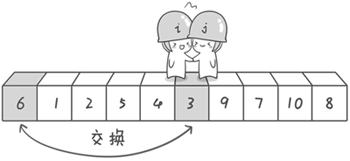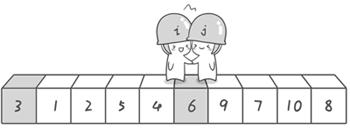### 递归

{3 1 2 5 4 6 9 7 10 8}

{3 1 2 5 4} 和 {9 7 10 8}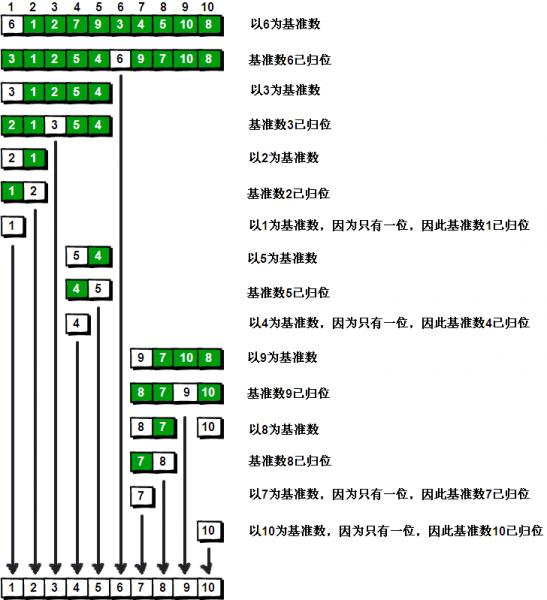# java 代码实现

## 核心代码实现

package com.github.houbb.sort.core.api;

import com.github.houbb.log.integration.core.Log;
import com.github.houbb.log.integration.core.LogFactory;
import com.github.houbb.sort.core.util.InnerSortUtil;

import java.util.List;

/**
* 快速排序
* @author binbin.hou
* @since 0.0.2
*/
public class QuickSort extends AbstractSort {

private static final Log log = LogFactory.getLog(QuickSort.class);

@Override
protected void doSort(List<?> original) {
this.quickSort(original, 0, original.size()-1);
}

/**
* 快速排序
* @param original 原始列表
* @param left 左边
* @param right 右边
* @since 0.0.2
*/
@SuppressWarnings("all")
private void quickSort(final List<?> original,
final int left,
final int right) {
if(left > right) {
return;
}

// 左右两边的哨兵指针
int leftIx = left;
int rightIx = right;
// 比较基准，直接取最左边的元素
Comparable basic = (Comparable) original.get(leftIx);

while (leftIx < rightIx) {
// 右边，从右向左移动找到第一个小于基准的数
while (leftIx < rightIx && InnerSortUtil.gte(original, rightIx, basic)) {
rightIx--;
}

// 左边，从左向右移动找到第一个大于基准的数
while (leftIx < rightIx && InnerSortUtil.lte(original, leftIx, basic)) {
leftIx++;
}

// 判断是否满足交换的条件
if(leftIx < rightIx) {
InnerSortUtil.swap(original, leftIx, rightIx);
}
}

// 更新基准的信息（i == j）
// 将最左边位置的元素，和此时的哨兵位置交换
InnerSortUtil.swap(original, left, leftIx);

// 执行递归调用
quickSort(original, left, leftIx-1);
quickSort(original, leftIx+1, right);
}

}


## InnerSortUtil 工具类

package com.github.houbb.sort.core.util;

import java.util.List;

/**
* 内部比较辅助类，可能会变更。
* 外部不要使用
* @author binbin.hou
* @since 0.0.2
*/
public final class InnerSortUtil {

/**
* 执行数据的交换
* @param original 原始
* @param i 第一个
* @param j 第二个
* @since 0.0.1
*/
@SuppressWarnings("unchecked")
public static void swap(List original,
int i, int j) {
Object temp = original.get(i);
original.set(i, original.get(j));
original.set(j, temp);
}

/**
* 是否大于等于元素
* @param original 原始
* @param i 索引
* @param target 指定元素
* @since 0.0.2
*/
@SuppressWarnings("all")
public static boolean gte(List original, int i, Comparable target) {
Comparable comparable = (Comparable) original.get(i);
return comparable.compareTo(target) >= 0;
}

/**
* 是否小于等于元素
* @param original 原始
* @param i 索引
* @param target 指定元素
* @since 0.0.2
*/
@SuppressWarnings("all")
public static boolean lte(List original, int i, Comparable target) {
Comparable comparable = (Comparable) original.get(i);
return comparable.compareTo(target) <= 0;
}

}


## 工具方法

/**
* 快速排序
* @param <T> 泛型
* @param list 列表
* @since 0.0.2
*/
public static <T extends Comparable<? super T>> void quick(List<T> list) {
Instances.singleton(QuickSort.class).sort(list);
}


# 代码测试

## 测试代码

List<Integer> list = Arrays.asList(6,1,2,7,9,3,4,5,10,8);
System.out.println("开始排序：" + list);
SortHelper.quick(list);
System.out.println("完成排序：" + list);


## 测试日志

### 数据交换时

// 判断是否满足交换的条件
if(leftIx < rightIx) {
InnerSortUtil.swap(original, leftIx, rightIx);
if(log.isDebugEnabled()) {
String info = String.format("l=%s, r=%s, lx=%s, rx=%s, 交换后：%s",
left, right, leftIx, rightIx, original);
log.debug(info);
}
} else {
if(log.isDebugEnabled()) {
String info = String.format("l=%s, r=%s, lx=%s, rx=%s, 无交换",
left, right, leftIx, rightIx);
log.debug(info);
}
}


### 基准归位时

// 更新基准的信息（i == j）
// 将最左边位置的元素，和此时的哨兵位置交换
InnerSortUtil.swap(original, left, leftIx);
if(log.isDebugEnabled()) {
String info = String.format("l=%s, lx=%s, 基准数归位：%s",
left, leftIx, original);
log.debug(info);
}


## 测试日志

开始排序：[6, 1, 2, 7, 9, 3, 4, 5, 10, 8]
l=0, r=9, lx=3, rx=7, 交换后：[6, 1, 2, 5, 9, 3, 4, 7, 10, 8]
l=0, r=9, lx=4, rx=6, 交换后：[6, 1, 2, 5, 4, 3, 9, 7, 10, 8]
l=0, r=9, lx=5, rx=5, 无交换
l=0, lx=5, 基准数归位：[3, 1, 2, 5, 4, 6, 9, 7, 10, 8]
l=0, r=4, lx=2, rx=2, 无交换
l=0, lx=2, 基准数归位：[2, 1, 3, 5, 4, 6, 9, 7, 10, 8]
l=0, r=1, lx=1, rx=1, 无交换
l=0, lx=1, 基准数归位：[1, 2, 3, 5, 4, 6, 9, 7, 10, 8]
l=0, lx=0, 基准数归位：[1, 2, 3, 5, 4, 6, 9, 7, 10, 8]
l=3, r=4, lx=4, rx=4, 无交换
l=3, lx=4, 基准数归位：[1, 2, 3, 4, 5, 6, 9, 7, 10, 8]
l=3, lx=3, 基准数归位：[1, 2, 3, 4, 5, 6, 9, 7, 10, 8]
l=6, r=9, lx=8, rx=9, 交换后：[1, 2, 3, 4, 5, 6, 9, 7, 8, 10]
l=6, r=9, lx=8, rx=8, 无交换
l=6, lx=8, 基准数归位：[1, 2, 3, 4, 5, 6, 8, 7, 9, 10]
l=6, r=7, lx=7, rx=7, 无交换
l=6, lx=7, 基准数归位：[1, 2, 3, 4, 5, 6, 7, 8, 9, 10]
l=6, lx=6, 基准数归位：[1, 2, 3, 4, 5, 6, 7, 8, 9, 10]
l=9, lx=9, 基准数归位：[1, 2, 3, 4, 5, 6, 7, 8, 9, 10]



# 开源地址

https://github.com/houbb/sort

Bubble_sort